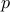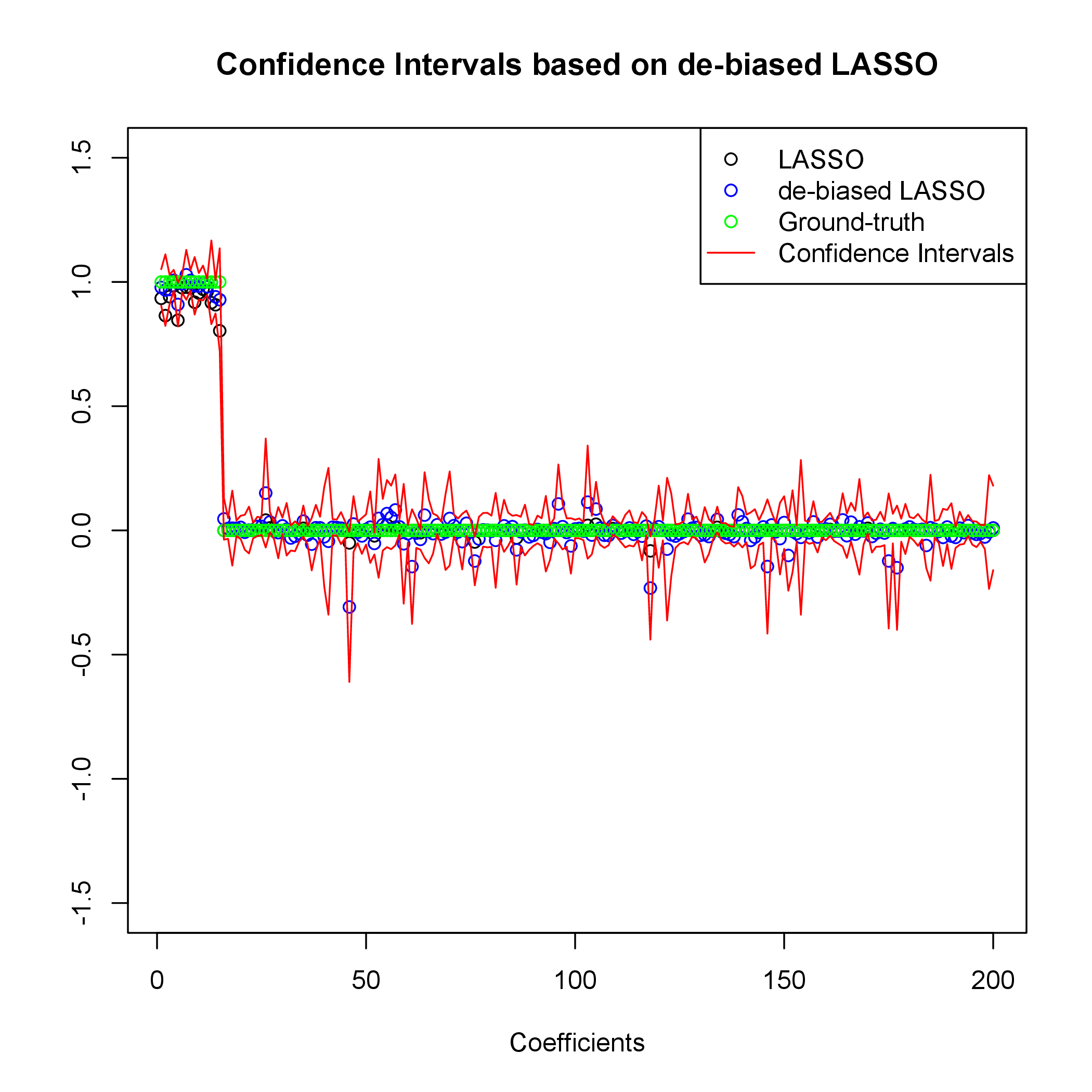# Confidence Intervals and Hypothesis Testing for High-Dimensional Regression

Overview: Fitting high-dimensional statistical models often requires the use of non-linear parameter estimation procedures. As a consequence, it is generally impossible to obtain an exact characterization of the probability distribution of the parameter estimates. This in turn implies that it is extremely challenging to quantify the uncertainty associated with a certain parameter estimate. Concretely, no commonly accepted procedure exists for computing classical measures of uncertainty and statistical significance as confidence intervals or-values for these models.

In our paper, we consider high-dimensional linear regression problem with sparse coefficients, and propose an efficient algorithm for constructing confidence intervals and-values for each single coefficient in the model. (See also our paper in NIPS 2013 that discusses generalizations of the same approach to generalized linear models, and regularized maximum likelihood estimation.)

This website provides an overview of our method along with the source code for its implementation. We would greatly appreciate feedback, in particular if you use our code. We are particularly interested in cases where the algorithm appears to fail. In this case please send us the data you use, or whatever needed to repeat your experiment.

Please send any feedback to: adelj at stanford.edu and/or montanari at stanford.edu.## Paper

Adel Javanmard and Andrea Montanari, Confidence Intervals and Hypothesis Testing for High-Dimensional Regression, 2013.